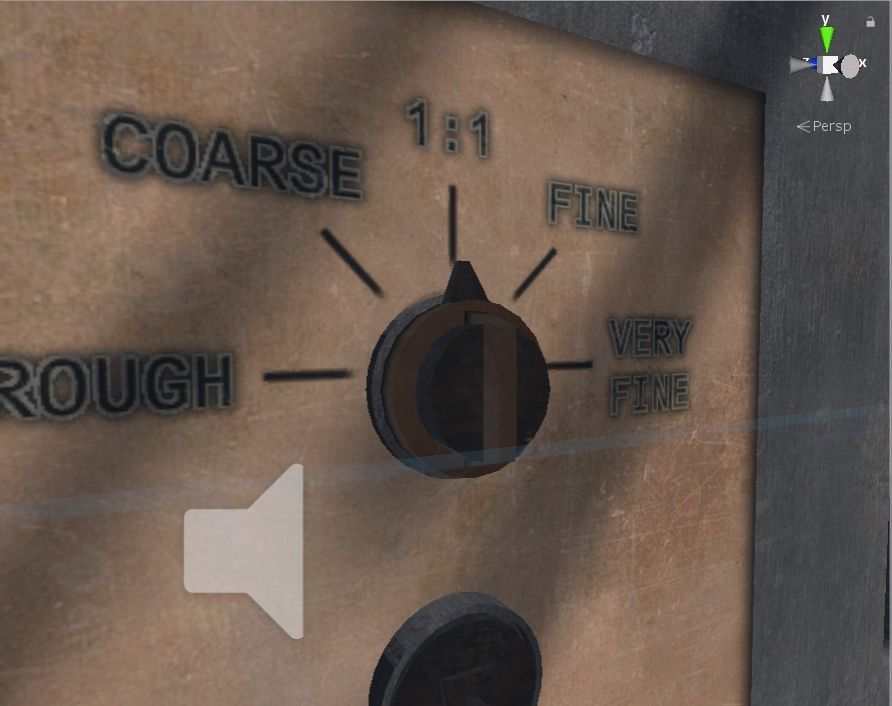# Rotate object based on rotation of SteamVR Controller?

I am trying to make a dial on a machine (similar to ones you may find on a stove), where the user can rotate it, but only on one axis (in this case, the Z axis) and cannot move it from its location. Here is an image of my dial:It should be noted that the scale of the model is 100, and it is a child of an empty GameObject whose scale is 1, which is a child of a model whose scale is 0.0075.

Here is the code I have so far:

``````using System.Collections;
using System.Collections.Generic;
using UnityEngine;
using Valve.VR.InteractionSystem;
[RequireComponent(typeof(Interactable))]
public class Dial914 : MonoBehaviour
{
Hand playerHand;
[SerializeField] Rigidbody dial;
// Use this for initialization
void Start()
{
}

private void HandAttachedUpdate()
{
dial.MoveRotation(Quaternion.Euler(0, 0, -playerHand.transform.rotation.z * transform.localScale.z)); //I actually don't know why we have to negate the hand rotation here, but it works
}

protected virtual void OnAttachedToHand(Hand hand)
{
playerHand = hand;
}
}
``````

This mostly works, but you can only rotate it halfway around and it starts to get buggy if you try to go further than that.

Here is a gfycat of the result:

``````Vector3 eulerRotation = new Vector3(0, 0, playerHand.transform.eulerAngles.z);